# 行星大气¶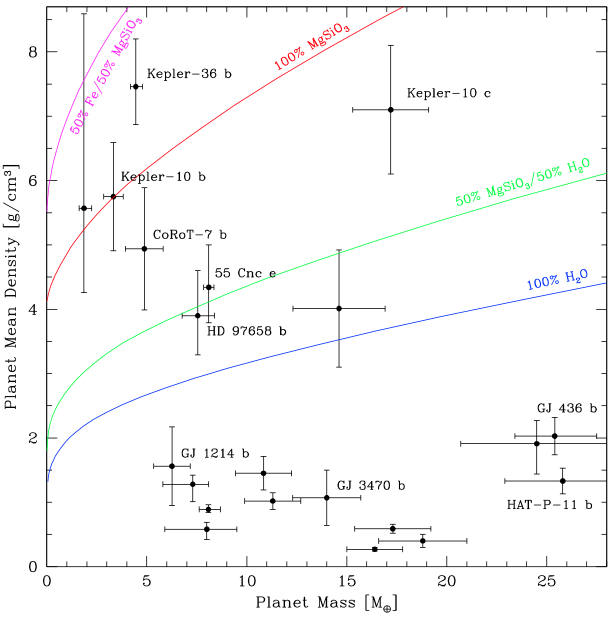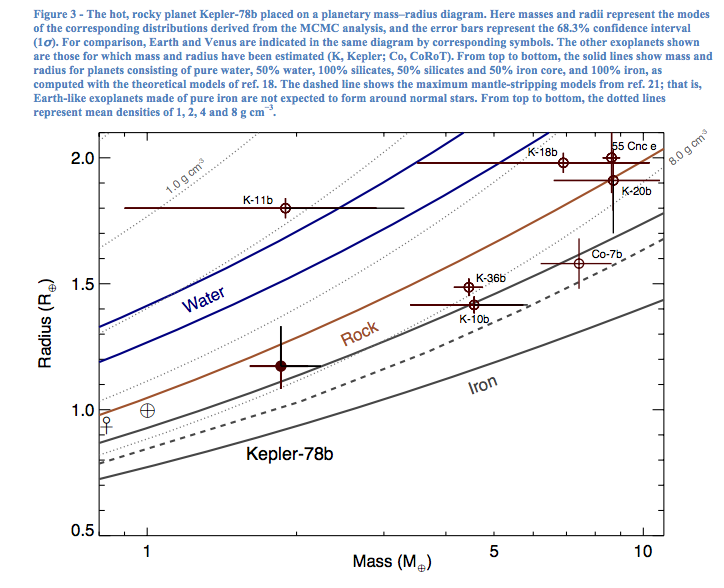## 基础知识¶

### 理解光谱¶

• 黑体辐射• 热平衡

• 辐射转移
• 光深度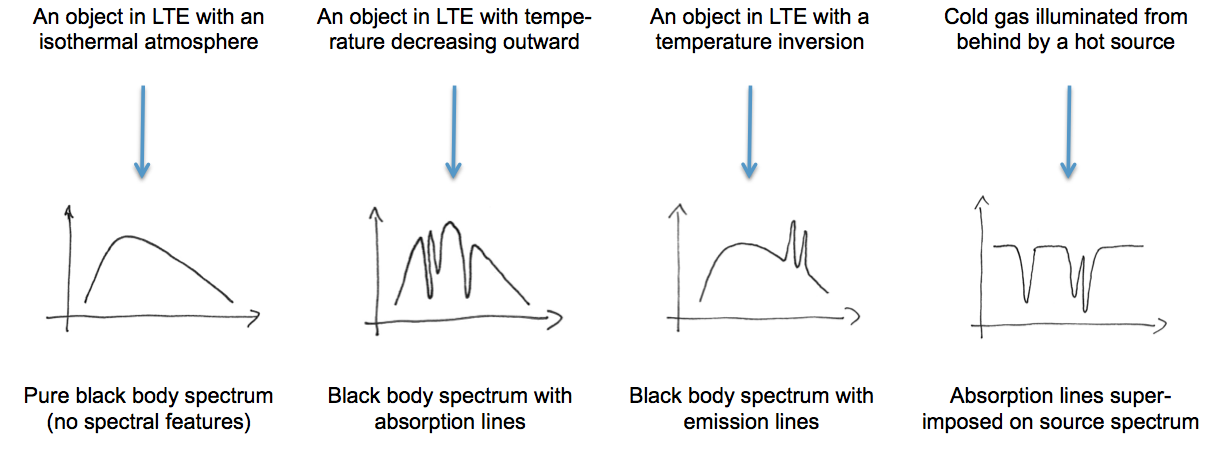### 化学成分¶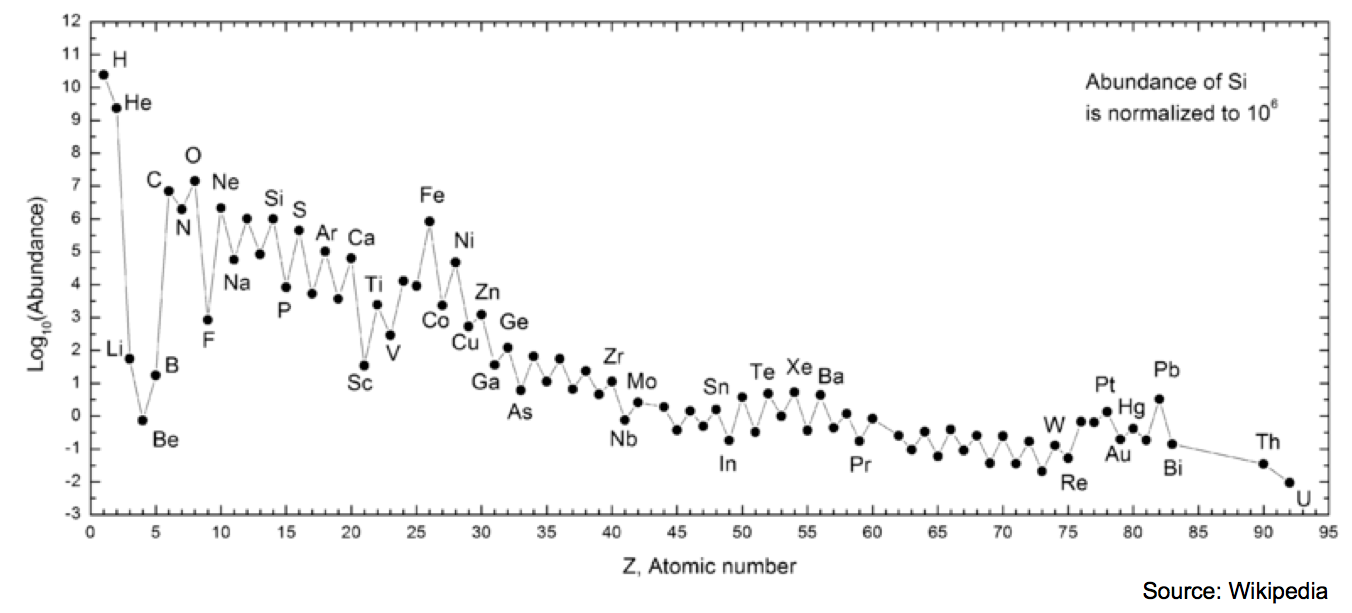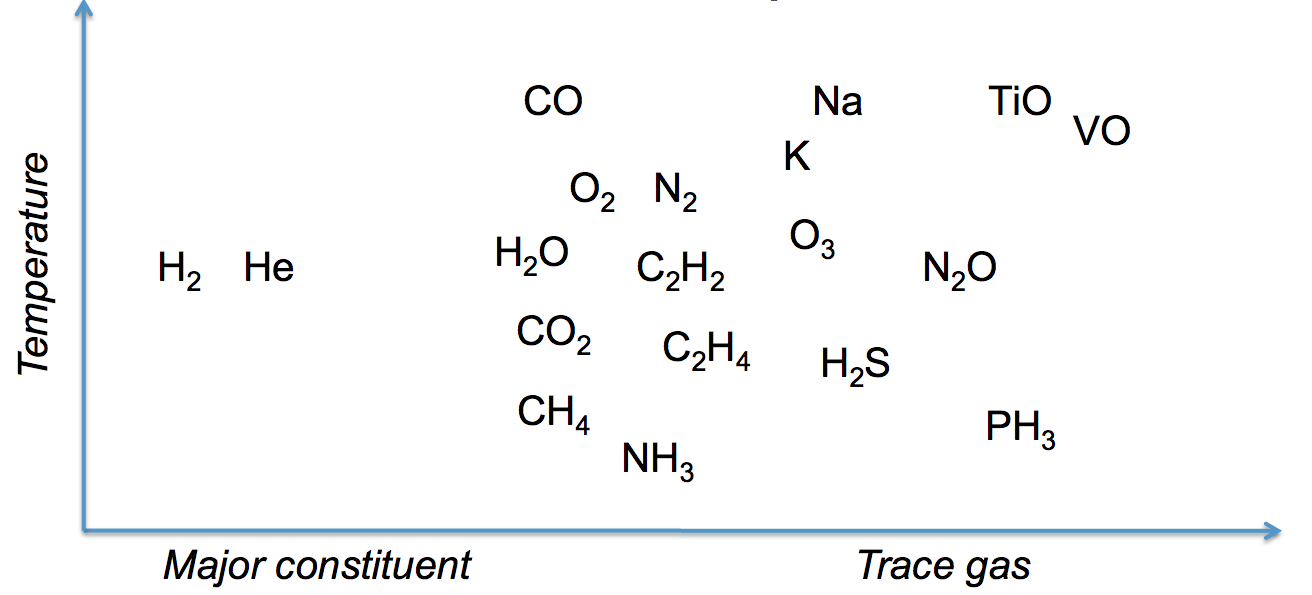1. 分子原子的电子跃迁会产生不同的谱线；
2. 分子的转动-振动谱线；
3. 散射，包括瑞利散射（散射粒子远小于光波长）和米散射（散射粒子大于光波长）。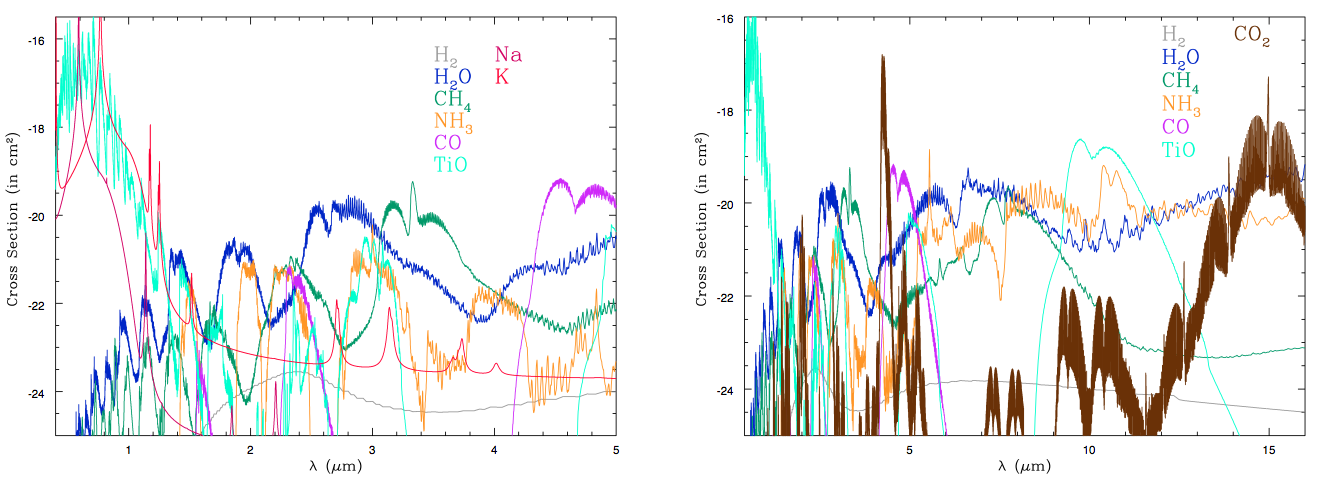### 压强-温度关系¶

$\frac{dP(z)}{dz} = -\rho g,$

$P = \frac{\rho}{\mu}kT.$

$P(z) = P_0 e^{-z/H},$

## 区分恒星光谱和行星光谱¶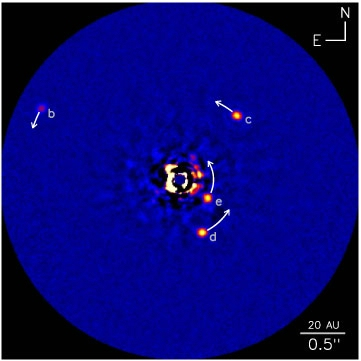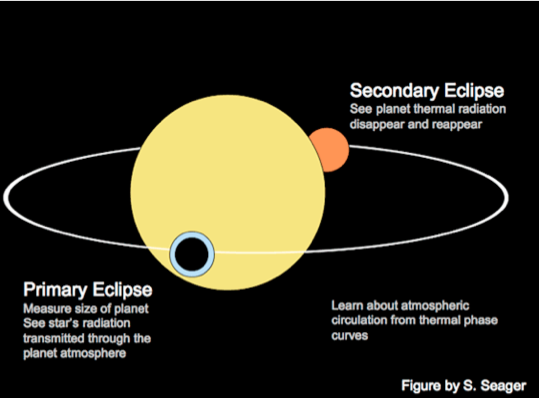### 透射光谱¶

$z(\lambda) = H (\ln \sigma(\lambda)+\text{\Const})$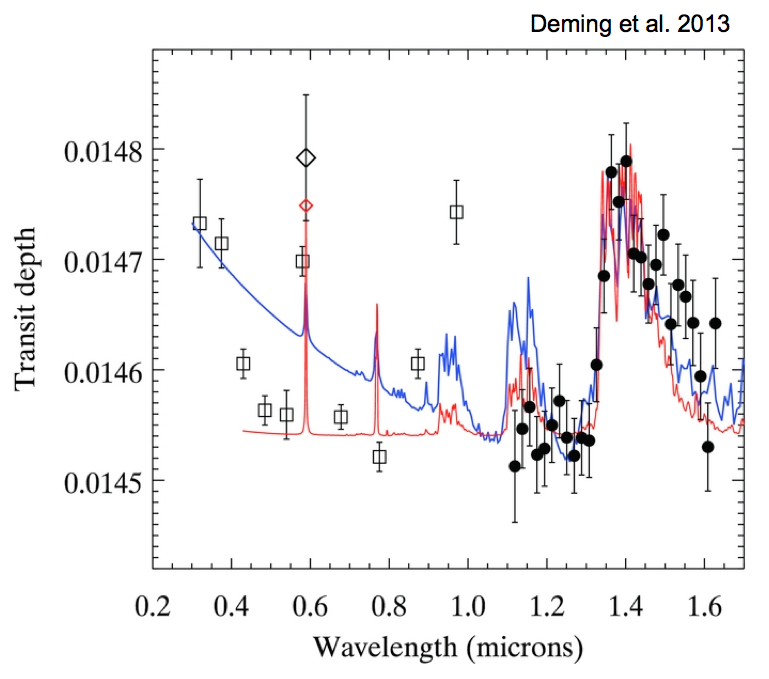HD 209458b， 右侧的巨大的峰值显示了水蒸气的透射波长。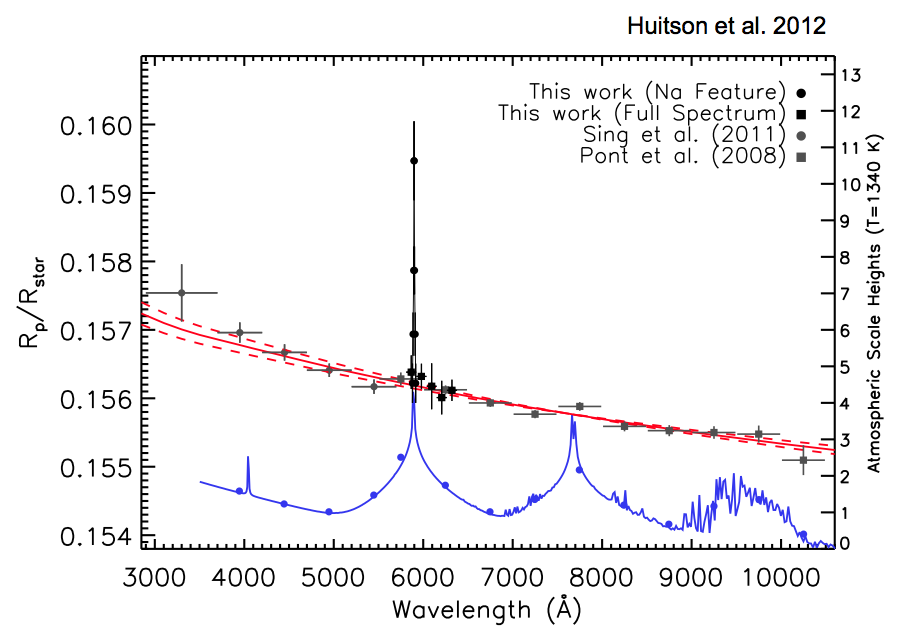HD 189733b，红线是观测结果，逐渐降低的半径显示了很可能有雾气或者云层导致瑞利散射。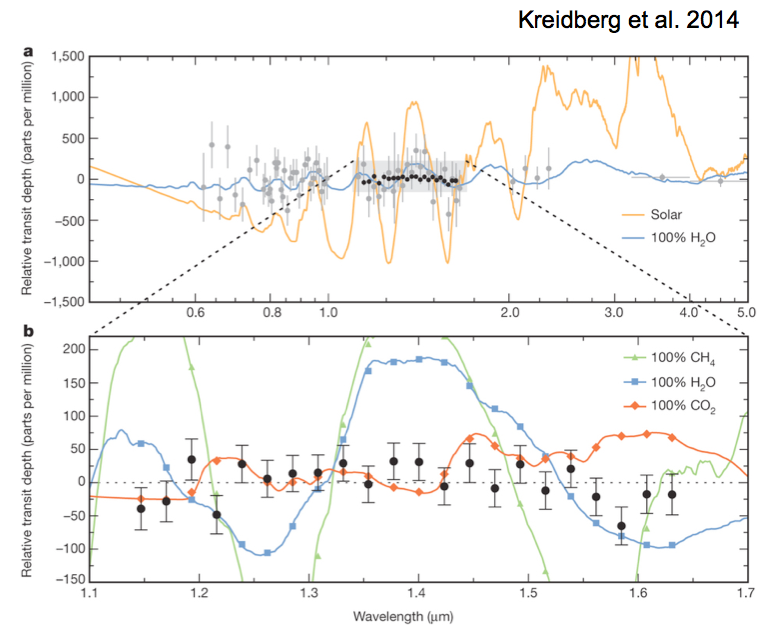GJ 1214b，几乎水平的结果暗示这颗行星有云层或者雾气。

### 热发射谱¶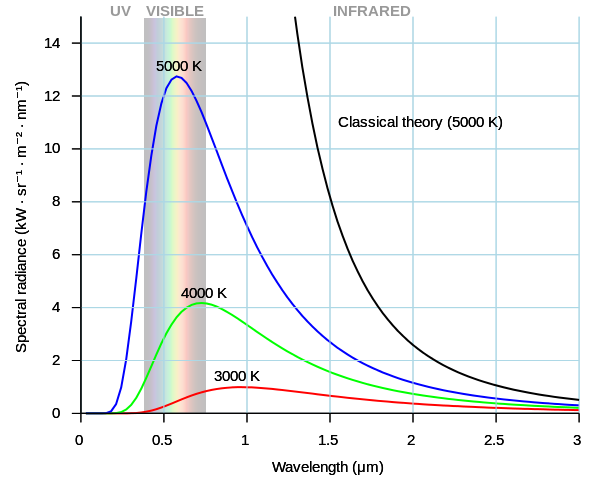$T_{eq} = T_{eff}\sqrt{R_s}{a}\left(\frac{1-A_B}{4}\right)^{1/4}.$

$\frac{F_p(\lambda)}{F_s(\lambda)} = \left(\frac{R_p}{R_s}\right)^2 \sqrt{R_s}{a}\left(\frac{1-A_B}{4}\right)^{/4}.$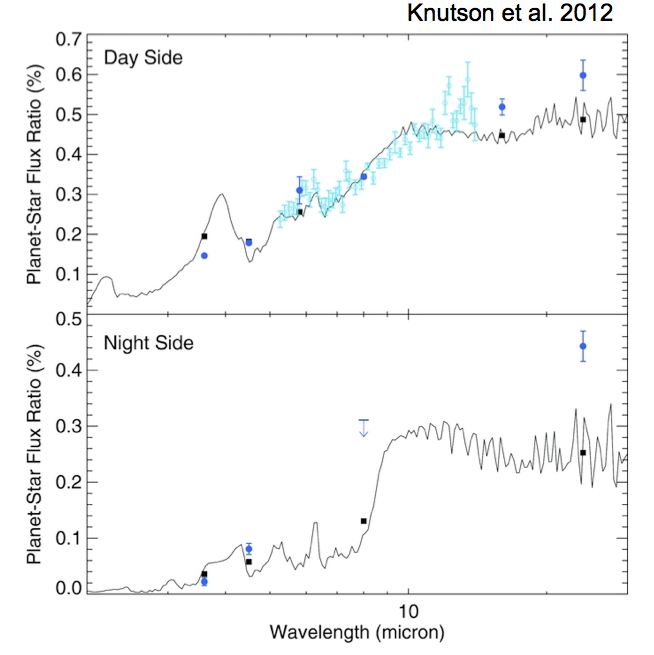HD 189733b，一颗被潮汐锁定的行星，下面一张图是夜晚部分的曲线，显然要比白天部分的要冷。

### 其他方式¶